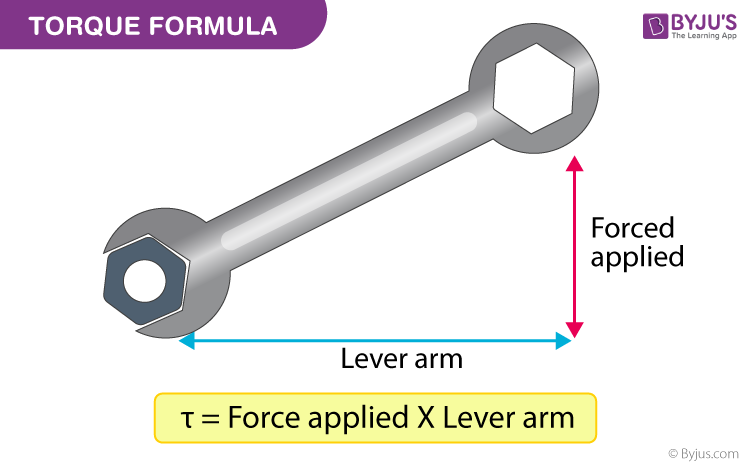# Torque Formula

When we take a wrench and fix a bolt; the force exerted alters the rotational moment along an axis. This is what is termed as Torque.Torque is described as the turning effect of force on the axis of rotation. In brief, it is a moment of force. It is characterized by τ.

Torque formula is articulated as

$$\begin{array}{l}\tau = F \times d\end{array}$$

Where,
Force applied = F
From the axis of rotation the perpendicular distance of force = d

The Magnitude of torque is articulated as

$$\begin{array}{l}\tau = Fd \ sin \ \theta \end{array}$$

Where θ is the angle between the force applied and the axis of rotation.
The SI unit for torque is Newton-meter (Nm).

## Torque Solved Examples

Underneath are some problems on torque which gives one an idea of how to calculate the terminologies related to torque.

Problem 1: The width of a door is 40 cm. If it is released by exerting a force of 2 N at its edge (away from the hinges).Compute the torque produced which causes the door to open.

Force applied = F = 2 N
Length of lever arm = d = 40 cm

Torque = 0.40 m (as distance amid the line of action of force and axis of rotation is 40 cm)

Torque = F × d
= 0.40 × 20
Torque = 8 Nm.

Problem 2: The Classroom door is of width 50 cm. If the Handle of the door is 20 cm from the edge and the Force of 5 N is applied on the handle. Compute the torque.